# Scatter, Box, and Violin Plots

lessR provides many versions of a scatter plot with its `Plot()` function for one or two variables with an option to provide a separate scatterplot for each level of one or two categorical variables. Access all scatterplots with the same simple syntax. The first variable listed without a parameter name, the `x` parameter, is plotted along the x-axis. Any second variable listed without a parameter name, the `y` parameter, is plotted along the y-axis. Each parameter may be represented by a continuous or categorical variable, a single variable or a vector of variables.

## The Data

Illustrate with the Employee data included as part of lessR.

``d <- Read("Employee")``
``````##
## >>> Suggestions
##
## Data Types
## ------------------------------------------------------------
## character: Non-numeric data values
## integer: Numeric data values, integers only
## double: Numeric data values with decimal digits
## ------------------------------------------------------------
##
##     Variable                  Missing  Unique
##         Name     Type  Values  Values  Values   First and last values
## ------------------------------------------------------------------------------------------
##  1     Years   integer     36       1      16   7  NA  7 ... 1  2  10
##  2    Gender character     37       0       2   M  M  W ... W  W  M
##  3      Dept character     36       1       5   ADMN  SALE  FINC ... MKTG  SALE  FINC
##  4    Salary    double     37       0      37   53788.26  94494.58 ... 56508.32  57562.36
##  5    JobSat character     35       2       3   med  low  high ... high  low  high
##  6      Plan   integer     37       0       3   1  1  2 ... 2  2  1
##  7       Pre   integer     37       0      27   82  62  90 ... 83  59  80
##  8      Post   integer     37       0      22   92  74  86 ... 90  71  87
## ------------------------------------------------------------------------------------------``````

As an option, lessR also supports variable labels. The labels are displayed on both the text and visualization output. Each displayed label consists of the variable name juxtaposed with the corresponding label. Create the table formatted as two columns. The first column is the variable name and the second column is the corresponding variable label. Not all variables need to be entered into the table. The table can be stored as either a `csv` file or an Excel file.

Read the variable label file into the l data frame, currently the only permissible name for the label file.

``l <- rd("Employee_lbl")``
``````##
## >>> Suggestions
##
## Data Types
## ------------------------------------------------------------
## character: Non-numeric data values
## ------------------------------------------------------------
##
##     Variable                  Missing  Unique
##         Name     Type  Values  Values  Values   First and last values
## ------------------------------------------------------------------------------------------
##  1     label character      8       0       8   Time of Company Employment ... Test score on legal issues after instruction
## ------------------------------------------------------------------------------------------``````

Display the available labels.

``l``
``````##                                                label
## Years                     Time of Company Employment
## Gender                                  Man or Woman
## Dept                             Department Employed
## Salary                           Annual Salary (USD)
## JobSat            Satisfaction with Work Environment
## Plan             1=GoodHealth, 2=GetWell, 3=BestCare
## Pre    Test score on legal issues before instruction
## Post    Test score on legal issues after instruction``````

## Continuous Variables

### Two Variables

A typical scatterplot visualizes the relationship of two continuous variables, here Years worked at a company, and annual Salary. Following is the function call to `Plot()` for the default visualization.

Because d is the default name of the data frame that contains the variables for analysis, the `data` parameter that names the input data frame need not be specified. That is, no need to specify `data=d`, though this parameter can be explicitly included in the function call if desired.

``Plot(Years, Salary)``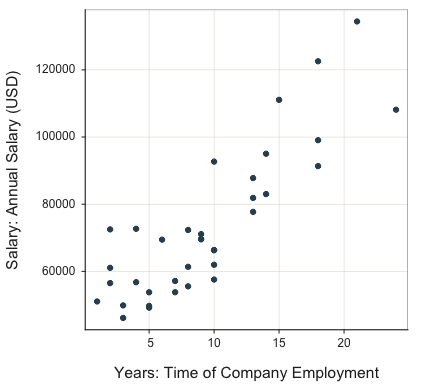``````## --- Pearson's product-moment correlation ---
##
## Years: Time of Company Employment
## Salary: Annual Salary (USD)
##
## Number of paired values with neither missing, n = 36
## Sample Correlation of Years and Salary: r = 0.852
##
## Hypothesis Test of 0 Correlation:  t = 9.501,  df = 34,  p-value = 0.000
## 95% Confidence Interval for Correlation:  0.727 to 0.923
## ``````

Enhance the default scatterplot with parameter `enhance`. The visualization includes the mean of each variable indicated by the respective line through the scatterplot, the 95% confidence ellipse, labeled outliers, least-squares regression line with 95% confidence interval, and the corresponding regression line with the outliers removed.

``Plot(Years, Salary, enhance=TRUE)``
``## [Ellipse with Murdoch and Chow's function ellipse from their ellipse package]``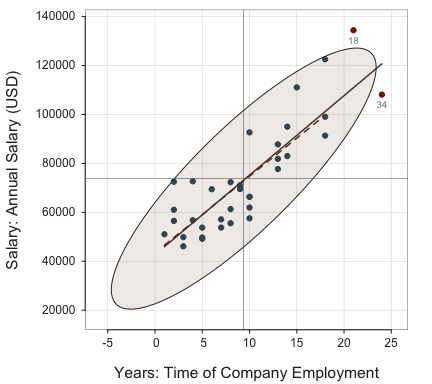``````## --- Pearson's product-moment correlation ---
##
## Years: Time of Company Employment
## Salary: Annual Salary (USD)
##
## Number of paired values with neither missing, n = 36
## Sample Correlation of Years and Salary: r = 0.852
##
## Hypothesis Test of 0 Correlation:  t = 9.501,  df = 34,  p-value = 0.000
## 95% Confidence Interval for Correlation:  0.727 to 0.923
##
##
## >>> Outlier analysis with Mahalanobis Distance
##
##   MD  ID
## ----- -----
## 8.14  18
## 7.84  34
##
## 5.63  31
## 5.58  19
## 3.75   4
## ...  ...``````

A variety of fit lines can be plotted. The available values: `"loess"` for general non-linear fit, `"lm"` for linear least squares, `"null"` for the null (flat line) model, `"exp"` for the exponential growth and decay, `"quad"` for the quadratic model, and `power` for the general power beyond 2. Setting `fit` to `TRUE` plots the `"loess"` line. With the value of `power`, specify the value of the root with parameter `fit_power`.

Here, plot the general non-linear fit. For emphasis set `plot_errors` to `TRUE` to plot the residuals from the line. The sum of the squared errors is displayed to facilitate the comparison of different models.

``Plot(Years, Salary, fit="loess", plot_errors=TRUE)``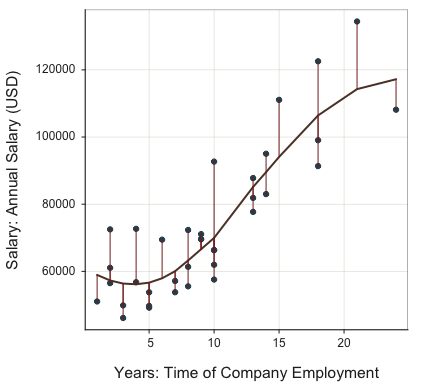``````##
## Fit: Mean Squared Error, MSE = 100,834,065
## ``````

Next, plot the exponential fit and show the residuals from the exponential curve. These data are approximately linear so the exponential curve does not vary far from a straight line. The function displays the corresponding sum of squared errors to assist in comparing various models to each other.

``Plot(Years, Salary, fit="exp", plot_errors=TRUE)``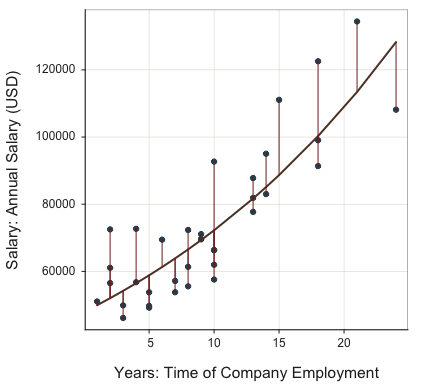``````##
##  Regressed linearized data of transformed data values of Salary with log()
##  For predicted values, back transform with exp() of regression model
##
##  Line: b0 = 10.777   b1 = 0.041    Fit: MSE = 0.022   Rsq = 0.722
## ``````

The parameter transforms the y variable to the specified power from the default of `1` before doing the regression analysis. The availability of this parameter provides for a wide range of modifications to the underlying functional form of the fit curve.

### Three Variables

Map a continuous variable, such as Pre, to the plotted points with the `size` parameter, a bubble plot.

``Plot(Years, Salary, size=Pre)``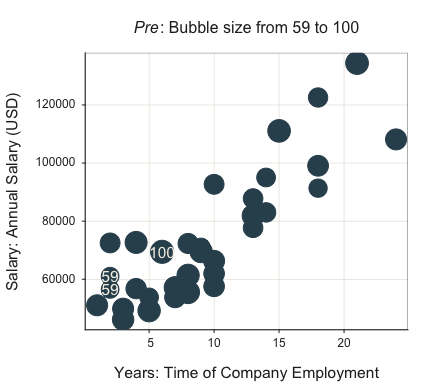``````## --- Pearson's product-moment correlation ---
##
## Years: Time of Company Employment
## Salary: Annual Salary (USD)
##
## Number of paired values with neither missing, n = 36
## Sample Correlation of Years and Salary: r = 0.852
##
## Hypothesis Test of 0 Correlation:  t = 9.501,  df = 34,  p-value = 0.000
## 95% Confidence Interval for Correlation:  0.727 to 0.923
##
##
## Some Parameter values (can be manually set)
## -------------------------------------------------------
## radius: 0.12    size of largest bubble
## power: 0.50     relative bubble sizes``````

Indicate multiple variables to plot along either axis with a vector defined according to the base R function `c()`. Plot the linear model for each variable according to the `fit` parameter set to `"lm"`. By default, when multiple lines are plotted on the same panel, the confidence interval is turned off by internally setting the parameter `fit_se` set to `0`. Explicitly override this parameter value as needed.

``Plot(c(Pre, Post), Salary, fit="lm", fit_se=0)``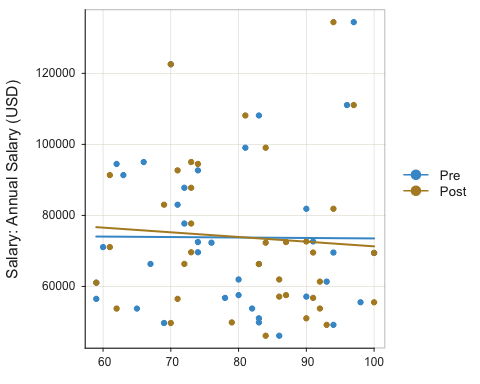``````## --- Pearson's product-moment correlation ---
##
## Post: Test score on legal issues after instruction
## Salary: Annual Salary (USD)
##
## Number of paired values with neither missing, n = 37
## Sample Correlation of Post and Salary: r = -0.070
##
## Hypothesis Test of 0 Correlation:  t = -0.416,  df = 35,  p-value = 0.680
## 95% Confidence Interval for Correlation:  -0.385 to 0.260
## ``````

### Scatterplot Matrix

Multiple variables for the first parameter value, `x`, and no values for `y`, plot as a scatterplot matrix. Pass a single vector, such as defined by `c()`. Request the non-linear fit line and corresponding confidence interval by specifying `TRUE` or `loess` for the `fit` parameter. Request a linear fit line with the value of `"lm"`.

``Plot(c(Salary, Years, Pre, Post), fit="lm")``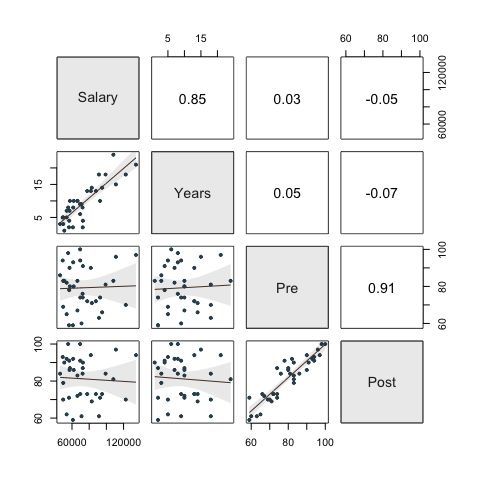### Smoothed and Binned Scatterplots

Smoothing and binning are two procedures for visualizing a relationship with many data values.

To obtain a larger data set, in this example generate random data with base R `rnorm()`, then plot. `Plot()` first checks the presence of the specified variables in the global environment (workspace). If not there, then from a data frame, of which the default value is d. Here, randomly generate values from normal populations for x and y in the workspace.

``````set.seed(13)
x=rnorm(4000)
y= 8*x + rnorm(4000,1, 30)
Plot(x, y)``````
``````## >>> Note: x is not in a data frame (table)
## >>> Note: y is not in a data frame (table)``````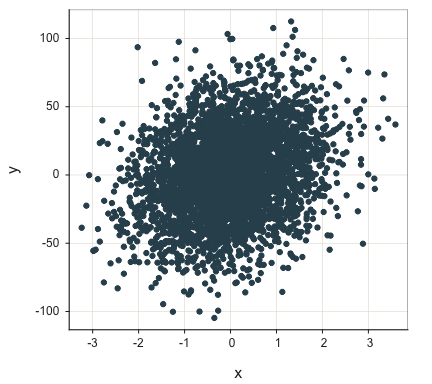``````## --- Pearson's product-moment correlation ---
##
## Number of paired values with neither missing, n = 4000
## Sample Correlation of x and y: r = 0.251
##
## Hypothesis Test of 0 Correlation:  t = 16.397,  df = 3998,  p-value = 0.000
## 95% Confidence Interval for Correlation:  0.222 to 0.280
## ``````

With large data sets, even for continuous variables there can be much over-plotting of points. One strategy to address this issue smooths the scatterplot by turning on the `smooth` parameter. The individual points superimposed on the smoothed plot are potential outliers. The default number of plotted outliers is 100. Turn off the plotting of outliers completely by setting parameter `smooth_points` to `0`. Show the linear trend with `fit` set to `"lm"`.

``Plot(x, y, smooth=TRUE, fit="lm")``
``````## >>> Note: x is not in a data frame (table)
## >>> Note: y is not in a data frame (table)``````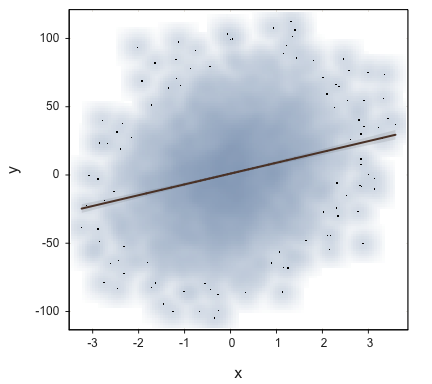``````## --- Pearson's product-moment correlation ---
##
## Number of paired values with neither missing, n = 4000
## Sample Correlation of x and y: r = 0.251
##
## Hypothesis Test of 0 Correlation:  t = 16.397,  df = 3998,  p-value = 0.000
## 95% Confidence Interval for Correlation:  0.222 to 0.280
##
##
##  Line: b0 = 1.030687568   b1 = 7.919636637    Fit: MSE = 917.032   Rsq = 0.063
## ``````

Another strategy for alleviating over-plotting makes the fill color mostly transparent with the `transparency` parameter, or turn off completely by setting `fill` to `"off"`. The closer the value of `trans` is to 1, the more transparent is the fill.

``Plot(x, y, transparency=0.95)``
``````## >>> Note: x is not in a data frame (table)
## >>> Note: y is not in a data frame (table)``````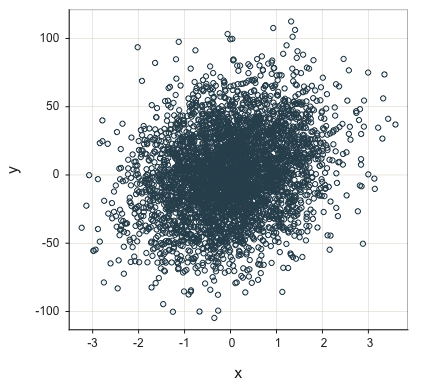``````## --- Pearson's product-moment correlation ---
##
## Number of paired values with neither missing, n = 4000
## Sample Correlation of x and y: r = 0.251
##
## Hypothesis Test of 0 Correlation:  t = 16.397,  df = 3998,  p-value = 0.000
## 95% Confidence Interval for Correlation:  0.222 to 0.280
## ``````

Another way to visualize a relationship when there are many data points is to bin the x-axis. Specify the number of bins with parameter `n_bins`. Plot() then computes the mean of y for each bin and connects the means by line segments. This procedure plots the conditional means by default without any assumption of form such as linearity. Specify the `stat` parameter for `median` to compute the median of y for each bin. The standard `Plot()` parameters `fill`, `color`, `size` and `segments` also apply.

``Plot(x, y, n_bins=5)``
``````## >>> Note: x is not in a data frame (table)
## >>> Note: y is not in a data frame (table)``````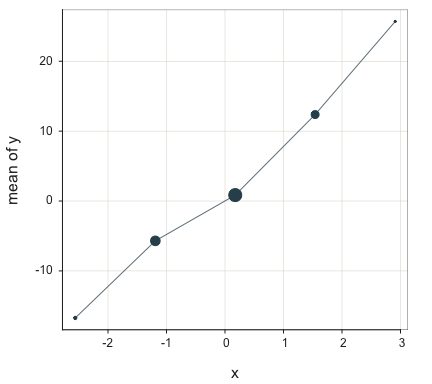``````##
## Table: Summary Stats
##
##                x          y
## -------  -------  ---------
## n           4000       4000
## n.miss         0          0
## min       -3.239   -104.740
## max        3.589    112.460
## mean      -0.003      1.006
##
##
## Table: mean of y for levels of x
##
##                   bin      n    midpt      mean
## ---  ----------------  -----  -------  --------
## 1     [-3.246,-1.873]    116   -2.560   -16.734
## 2     (-1.873,-0.508]   1090   -1.191    -5.699
## 3      (-0.508,0.858]   2001    0.175     0.848
## 4       (0.858,2.223]    743    1.541    12.374
## 5       (2.223,3.596]     50    2.909    25.696``````

### One Variable

The default plot for a single continuous variable includes not only the scatterplot, but also the superimposed violin plot and box plot, with outliers identified. Call this plot the VBS plot.

``Plot(Salary)``
``````## [Violin/Box/Scatterplot graphics from Deepayan Sarkar's lattice package]
##
## >>> Suggestions
## Plot(Salary, out_cut=2, fences=TRUE, vbs_mean=TRUE)  # Label two outliers ...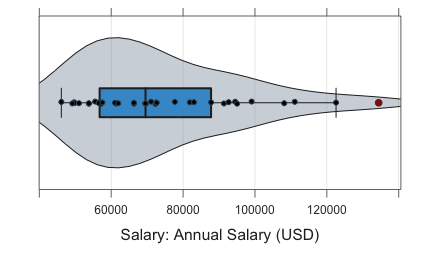``````## --- Salary ---
## Present: 37
## Missing: 0
## Total  : 37
##
## Mean         : 73795.557
## Stnd Dev     : 21799.533
## IQR          : 31012.560
## Skew         : 0.190   [medcouple, -1 to 1]
##
## Minimum      : 46124.970
## Lower Whisker: 46124.970
## 1st Quartile : 56772.950
## Median       : 69547.600
## 3rd Quartile : 87785.510
## Upper Whisker: 122563.380
## Maximum      : 134419.230
##
##
## --- Outliers ---     from the box plot: 1
##
## Small      Large
## -----      -----
##             134419.23
##
## Number of duplicated values: 0
##
## Parameter values (can be manually set)
## -------------------------------------------------------
## size: 0.61      size of plotted points
## out_size: 0.82  size of plotted outlier points
## jitter_y: 0.45 random vertical movement of points
## jitter_x: 0.00  random horizontal movement of points
## bw: 9529.04       set bandwidth higher for smoother edges``````

Control the choice of the three superimposed plots – violin, box, and scatter – with the `vbs_plot` parameter. The default setting is `"vbs"` for all three plots. Here, for example, obtain just the box plot. Or, use the alias `BoxPlot()` in place of `Plot()`.

``Plot(Salary, vbs_plot="b")``
``````## [Violin/Box/Scatterplot graphics from Deepayan Sarkar's lattice package]
##
## >>> Suggestions
## Plot(Salary, out_cut=2, fences=TRUE, vbs_mean=TRUE)  # Label two outliers ...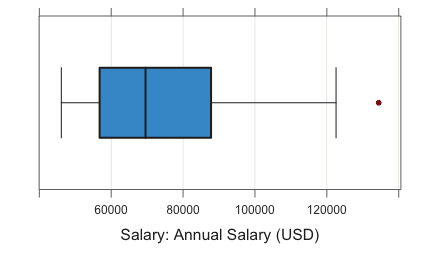``````## --- Salary ---
## Present: 37
## Missing: 0
## Total  : 37
##
## Mean         : 73795.557
## Stnd Dev     : 21799.533
## IQR          : 31012.560
## Skew         : 0.190   [medcouple, -1 to 1]
##
## Minimum      : 46124.970
## Lower Whisker: 46124.970
## 1st Quartile : 56772.950
## Median       : 69547.600
## 3rd Quartile : 87785.510
## Upper Whisker: 122563.380
## Maximum      : 134419.230
##
##
## --- Outliers ---     from the box plot: 1
##
## Small      Large
## -----      -----
##             134419.23
##
## Number of duplicated values: 0``````

### Cleveland Dot Plot

Create a Cleveland dot plot when one of the variables has unique (ID) values. In this example, for a single variable, row names are on the y-axis. The default plots sorts by the value plotted with the default value of parameter `sort_yx` of `"+"` for an ascending plot. Set to `"-"` for a descending plot and `"0"` for no sorting.

``Plot(Salary, row_names)``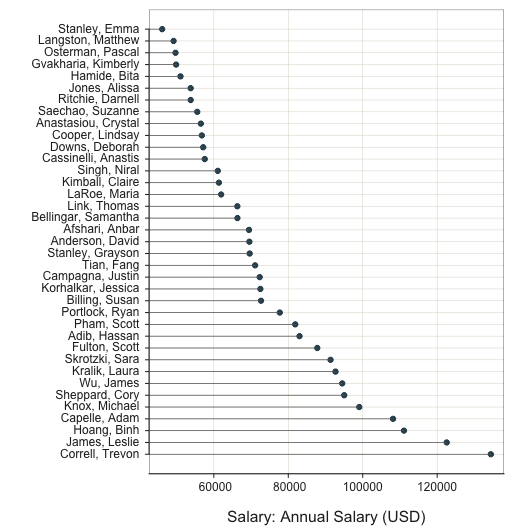``````##
## --- Salary ---
##
##      n   miss      mean        sd       min       mdn       max
##      37      0   73795.6   21799.5   46125.0   69547.6  134419.2
##
##
## --- Outliers ---     from the box plot: 1
##
## Small      Large
## -----      -----
##             134419.2
##
## Some Parameter values (can be manually set)
## -------------------------------------------------------
## size: 0.80  size of plotted points
## jitter_y: 0.00  random vertical movement of points
## jitter_x: 0.00  random horizontal movement of points``````

The standard scatterplot version of a Cleveland dot plot follows, with no sorting and no line segments.

``Plot(Salary, row_names, sort_yx="0", segments_y=FALSE)``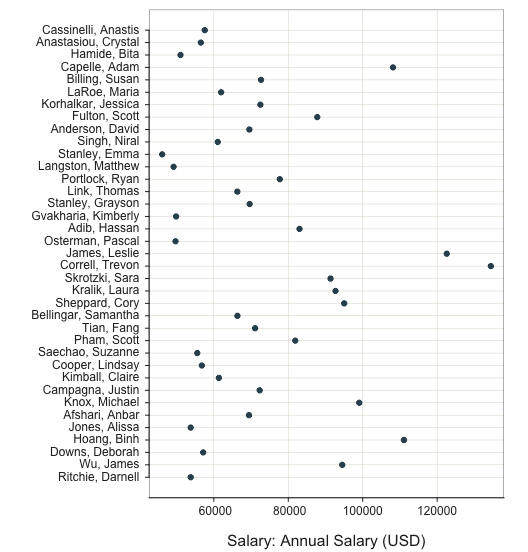``````##
## --- Salary ---
##
##      n   miss      mean        sd       min       mdn       max
##      37      0   73795.6   21799.5   46125.0   69547.6  134419.2
##
##
## --- Outliers ---     from the box plot: 1
##
## Small      Large
## -----      -----
##             134419.2
##
## Some Parameter values (can be manually set)
## -------------------------------------------------------
## size: 0.80  size of plotted points
## jitter_y: 0.00  random vertical movement of points
## jitter_x: 0.00  random horizontal movement of points``````

This Cleveland dot plot has two x-variables, indicated as a standard R vector with the `c()` function. In this situation, the two points on each row are connected with a line segment. By default the rows are sorted by distance between the successive points.

``Plot(c(Pre, Post), row_names)``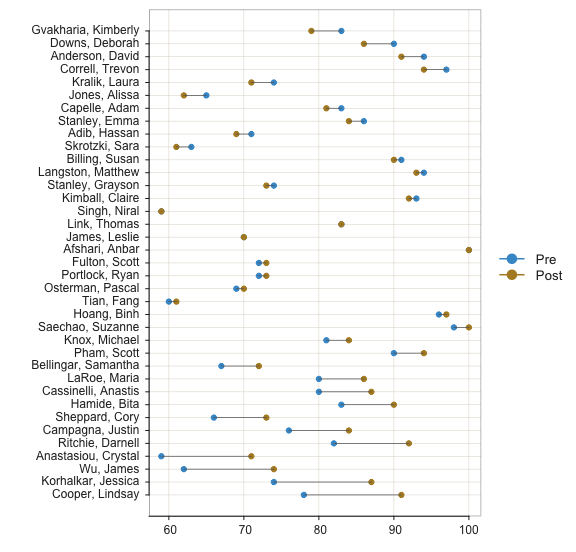``````##
## --- Pre ---
##
##      n   miss    mean      sd     min     mdn     max
##      37      0    78.8    12.0    59.0    80.0   100.0
##
##
## --- Post ---
##
##      n   miss    mean      sd     min     mdn     max
##      37      0    81.0    11.6    59.0    84.0   100.0
##
## Some Parameter values (can be manually set)
## -------------------------------------------------------
## size: 0.80  size of plotted points
## jitter_y: 0.00  random vertical movement of points
## jitter_x: 0.00  random horizontal movement of points``````

## Categorical and Continuous Variables

A mixture of categorical and continuous variables can be plotted a variety of ways, as illustrated below.

### Two Continuous, One Categorical

Plot a scatterplot of two continuous variables for each level of a categorical variable on the same panel with the `by` parameter. Here, plot Years and Salary each for the two levels of Gender in the data. Colors and geometric plot shapes can distinguish between the plots. For all variables except an ordered factor, the default plots according to the default qualitative color palette, `"hues"`, with the geometric shape of a point.

``Plot(Years, Salary, by=Gender)``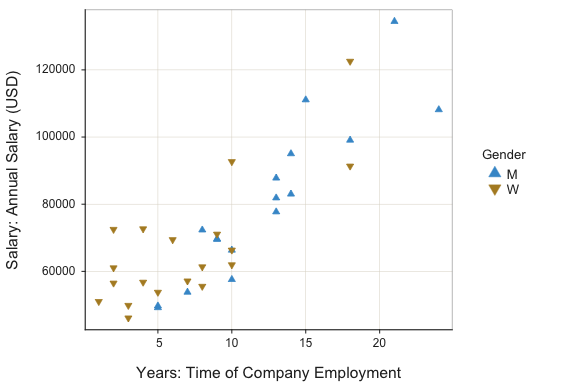Change the plot colors with the `fill` (interior) and `color` (exterior or edge) parameters. Because there are two levels of the `by` variable, specify two fill colors and two edge colors each with an R vector defined by the `c()` function. Also, include the regression line for each group with the `fit` parameter and increase the size of the plotted points with the `size` parameter.

``````Plot(Years, Salary, by=Gender, size=2, fit="lm",
fill=c("olivedrab3", "gold1"),
color=c("darkgreen", "gold4")
)``````